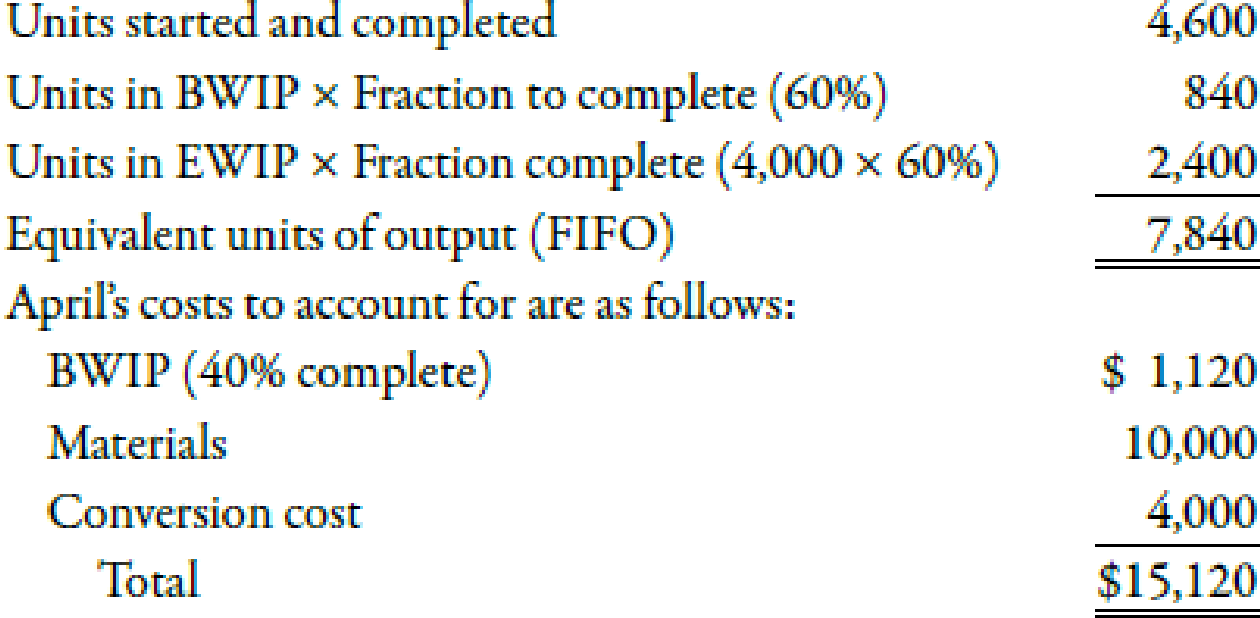Chapter 6, Problem 55E### Managerial Accounting: The Corners...

7th Edition
Maryanne M. Mowen + 2 others
ISBN: 9781337115773

#### Solutions

Chapter
Section### Managerial Accounting: The Corners...

7th Edition
Maryanne M. Mowen + 2 others
ISBN: 9781337115773
Textbook Problem
9 views

# (Appendix 6A) First-In, First-Out Method; Unit Cost; Valuing InventoriesLoren Inc. manufactures products that pass through two or more processes. During April, equivalent units were computed using the FIFO method:Required: 1. Calculate the unit cost for April using the FIFO method. (Note: Round to two decimal places.) 2. Using the FIFO method, determine the cost of EWIP and the cost of the goods transferred out.

1.

To determine

Compute unit cost of Company LI using FIFO method.

Explanation

First-in first out method:

A method which separates the beginning inventory units from units produced during a period is known as FIFO method. In FIFO method, only cost incurred during a period is considered to calculate unit cost.

Use the following formula to calculate unit cost:

Unit cost=Material cost+Conversion cost Equivalent�

2.

To determine

Calculate the cost of EWIP and cost of goods transferred out by applying FIFO method.

### Still sussing out bartleby?

Check out a sample textbook solution.

See a sample solution

#### The Solution to Your Study Problems

Bartleby provides explanations to thousands of textbook problems written by our experts, many with advanced degrees!

Get Started

#### Find more solutions based on key concepts